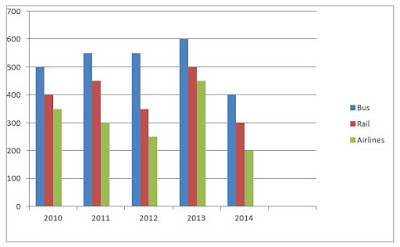### Data Interpretation for Bank Exams

Directions (Q.1-5): Study the following bar graph carefully and answer the questions given below:

The graph shows the no. of persons using different modes of transport over the years.1).What is the difference between the total number of persons travelling by Airlines in 2011, 2013 and 2014 and that travelling by Rail from 2010 and 2012?
a) 100
b) 200
c) 300
d) 350
e) None of these

2).In 2012 the number of persons travelling by Bus is approximately what per cent of the number of persons travelling by Bus, Rail and Airlines together in that year?
a) 42.82%
b) 48%
c) 68%
d) 57.82%
e) None of these

3).What is the total number of persons travelling by Airlines in all the years together?
a) 1600
b) 1400
c) 1500
d) 1450
e) 1550

4).What is the ratio of the number of persons travelling by Airlines in 2011 and 2012 together to the number of persons travelling by Bus in 2014?

a) 11:80
b) 11:2
c) 11:8
d) 10:8
e) None of these

5).What is the average number of persons travelling by Bus in all the years together?
a) 420
b) 320
c) 510
d) 520
e) 620

Directions (Q.6-10): Study the bar graph carefully to answer the questions that follow:

Profit (in RS. lakh) earned by three companies over the years.6).The profit earned by Company B in the year 2011 was what per cent of the total profit earned by all the three companies in that year?
a) 40%
b) 38%
c) 35%
d) 46%
e) None of these

7).If the income of Company C in the year 2012 was RS.5328925 what was its expenditure in that year?
a) RS.692895
b) RS. 728925
c) RS. 828925
d) RS. 538925
e) None of these

8).What is the average profit earned by Company A in all the years together?
a) RS.4350000
b) RS.4000000
c) RS.3864920
d) RS.6838450
e) RS.5124000

9).What was the percentage increase in the profit of Company B in the year 2009 from the previous year?
a) 67%
b) 58%
c) 78%
d) 82%
e) None of these

10).If the expenditure of Company A in the year 2013 was RS.1583259, what was the income in that year?
a) RS.5963218
b) RS.6985324
c) RS.4328690
d) RS.7083259
e) None of these

1). b)
2).b )
3). e)
4).e )
5).d )
6). a)
7). c)
8). b)
9).e )
10). d)

Solutions

2). Required %= (550/1150)×100=47.82%≈48%

3). Total number of persons travelling in all the years together by Airlines=350+300+250+450+200=1550

4). Required ratio =550: 400=11:8

5). Average number of persons travelling by buses in all the years together= (500+550+550+600+400)/5=2600/5=520

6). Profit of Company B=RS.60 lakh in 2011. And profit of three companies in that year=50+60+40=150
∴ Required %= (60/150)×100=40%

7). Expenditure of Company C=Income-Profit=5328925-4500000=RS.828925